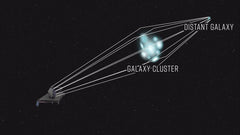# Theory of General Relativity

## Gravity as Curvature of Space-Time

,gravity is not seen as a force that pulls objects together, but rather as a result of the curvature of space and time caused by the presence of mass and energy. This curvature can be thought of like a fabric or a rubber sheet that is warped or stretched by the presence of an object with mass or energy.

To understand this concept better, imagine a flat rubber sheet representing empty space. Now, place a heavy ball in the center of the sheet, and watch as it creates a dip or a curve in the rubber sheet. This represents how the mass of the ball warps the fabric of space and time around it. If you place a smaller ball or any other object on the sheet near the heavy ball, it will roll or be pulled towards the dip in the rubber sheet created by the heavy ball.

This is similar to how gravity works in space-time. The mass of an object creates a "dent" or "bump" in the fabric of space-time, and other objects are attracted towards it. The larger the mass of an object, the greater the curvature of space-time around it, and the stronger the gravitational pull it exerts on other objects.

## here are three more examples to illustrate the concept of mass warping space-time:

• ### The Sun and the planets:

The Sun has a very large mass, which warps the space-time around it. This creates a gravitational field that pulls the planets towards it, causing them to orbit the Sun. The planets are attracted to the "dent" or "bump" in the fabric of space-time created by the Sun's mass.
• ### Black holes:

Black holes are objects with an extremely large mass that are so dense that they warp space-time to an extreme degree. They create a "dent" in space-time that is so deep that anything that gets too close to the black hole will be pulled towards it with an incredible force. The gravitational pull of a black hole is so strong that not even light can escape from it.

• ### Gravitational lensing:The curvature of space-time can also cause light to bend as it passes near an object with a large mass. This effect is known as gravitational lensing. For example, when light from a distant star passes near a massive galaxy, the space-time around the galaxy bends the light, causing it to bend and curve around the galaxy. This can create a distorted or magnified image of the distant star, allowing astronomers to study it in more detail.

Einstein & Maxwell equations

Einstein was heavily influenced by the work of Maxwell and his equations. In fact, his development of the theory of relativity was built upon Maxwell's equations and their implications for the nature of space and time.

Maxwell's equations describe the behavior of electromagnetic waves, which includes visible light. Einstein used this understanding to develop his ideas about the nature of light and its propagation through space. In particular, he used the equations to explain the behavior of light in his theory of special relativity.

Einstein also used Maxwell's equations to develop his theory of general relativity, which describes the behavior of gravity in terms of the curvature of space and time. This theory was based on the idea that mass and energy warp the fabric of space-time, which is similar to the way that electric charge affects the behavior of electric and magnetic fields according to Maxwell's equations.

Overall, Einstein's work built on Maxwell's equations and extended our understanding of the fundamental laws of physics.

The four equations are:

1. Gauss's law for electric fields: This equation states that the electric flux through a closed surface is proportional to the electric charge enclosed within that surface.

2. Gauss's law for magnetic fields: This equation states that the magnetic flux through a closed surface is always zero.

3. Faraday's law of electromagnetic induction: This equation states that a changing magnetic field induces an electric field.

4. Ampere's law with Maxwell's correction: This equation states that a changing electric field can create a magnetic field. Maxwell's correction to Ampere's law involves the addition of a term that accounts for the displacement current, which is the flow of electric charge in a changing electric field.

Together, these four equations describe the behavior of electric and magnetic fields in a unified way and explain how they interact with one another. They are used to predict and explain a wide range of physical phenomena, such as the behavior of light, the properties of electromagnetic waves, and the behavior of electric and magnetic fields in various materials.

\$11.00 \$17.65